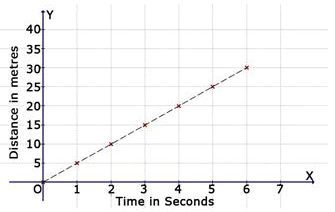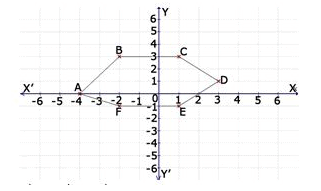Courses

Test: Introduction To Graphs- 1

10 Questions MCQ Test Mathematics (Maths) Class 8 | Test: Introduction To Graphs- 1

Description
Attempt Test: Introduction To Graphs- 1 | 10 questions in 10 minutes | Mock test for Class 8 preparation | Free important questions MCQ to study Mathematics (Maths) Class 8 for Class 8 Exam | Download free PDF with solutions
QUESTION: 1

In which quadrant does the point P (-4, 1) lie?

Solution: It is because 2nd quadrant Xi axis consist of negative number and Y axis has positive numbers .i.e. (-,+)
QUESTION: 2

On which axis does the point (-1, 0) lie?

Solution:

the point (-1, 0) lie on X axis as the Y value is 0 .

QUESTION: 3

Find the distance covered in 2 seconds.Solution: 10m is the correct answer because in the graph at 2 seconds, the line is on 10m.
QUESTION: 4

Find the distance covered in 5 seconds.Solution:
QUESTION: 5

Find the distance covered in 7 seconds.Solution:
QUESTION: 6

Find the coordinates of the point B from the graph.Solution:

The point is -2 units from x-coordinate and 3 units from y coordinate, hence the coordinates of the point is (-2,3).

QUESTION: 7

Find the distance covered in 6 seconds.Solution:
QUESTION: 8

Find the distance covered in 4 seconds.Solution:
QUESTION: 9

Find the coordinates of the point A from the graph.Solution:
QUESTION: 10

Find the distance covered in 3 seconds.Solution: As in this graph it shows that distance covered in 3 see second is 15 meter.Use Code STAYHOME200 and get INR 200 additional OFF Use Coupon Code

Track your progress, build streaks, highlight & save important lessons and more!

Similar ContentRelated tests